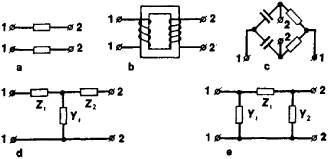# Four-Terminal Network

## Four-Terminal Network

an electrical network or a part of a network with four poles, or terminals, to which other networks or parts of another network may be connected. The four-terminal network is the most widely used type of multiterminal network.Figure 1. Schematics of four-terminal networks: (a) a power line, (b) a transformer, (c) a bridge circuit, (d) a four-terminal T network, (e) a four-terminal pi network; (1) input terminals, (2) output terminals, (Z) impedances of the network elements, (Y) admittances of the network elements

In four-terminal networks, two pairs of terminals, called the input terminals and the output terminals, are usually distinguished (Figure 1). The input terminals are used to connect a network to a source of electrical energy; the output terminals, to connect a network to a load. If the four terminals are grouped in pairs, the network is known as a two-terminal-pair network, which is also called a four-pole, a quadripole, a four-pole network, a quadripole network, or a quadrupole network.

If the relationship between the currents flowing through a four-terminal network and the voltages across the network’s terminals is linear, the network is said to be linear; in the opposite case, the network is referred to as nonlinear. The main characteristic of a linear four-terminal network is the complex transfer ratio, which is equal to the ratio of the complex amplitudes at the network’s input and output. A four-terminal network is said to be bilateral if its characteristics do not change when the connections to a source and a detector are reversed.

Examples of four-terminal networks include transformers, power lines, bridge circuits, and electric filters.

Site: Follow: Share:
Open / Close